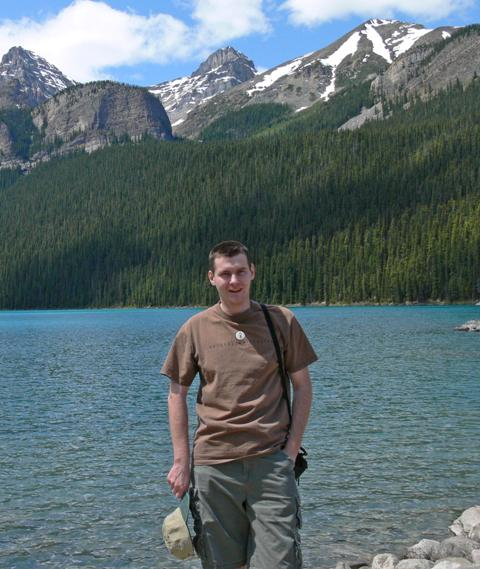Csaba D. Tóth Department of Mathematics California State University Northridge Room SN 434, 18111 Nordhoff St. Northridge, CA 91330-8313 Phone: (818) 677-2826 csaba.toth ♠ csun.edu Handbook of Discrete and Computational Geometry, 3rd edition (J.E. Goodman, J. O'Rourke, and C.D. Tóth, eds.) CRC Press LLC, Boca Raton, FL, November 13, 2017. New trends from Classical Theorems in Geometry, Combinatorics, and Topology June 4-9, 2023, Casa Matemática Oaxaca, Mexico. 39th International Symposium on Computational Geometry (SoCG) June 12-15, 2023, Richardson, TX, USA. 49th Workshop on Graph-Theoretic Concepts in Computer Science (WG) June 28-30, 2023, Fribourg, Switzerland. Algorithms and Data Structures Symposium (WADS) July 31-August 2, 2023, Montréal, QC, Canada. 31st Symposium on Graph Drawing and Network Visualization (GD) September 20–22, 2023, Isola delle Femmine, Italy. Discrete Geometry and Convexity Aug-Dec, 2023, Erdős Center, Budapest, Hungary. Publications:     in chronological order     sorted by types Manuscripts Courses: 2023: Math 262, Math 501 2022: Math 262, Math 320, Math 450A, Math 552 2021: Math 262, Math 320, Math 462, Math 552, Math 651C 2020: Math 326, Math 462, Math 499C 2019: Math 326 (Discrete Mathematics), Math 501 (Topology) 2018: Math 366 (Combinatorics), Math 494 2017: Math 320 (Foundations of Higher Mathematics), Math 482 2016: Math 366, Math 501, Math 589, Math 482 2015: Math 450 2014: Math 262, Math 482 2013: Math 326 (Discrete Mathematics), Math 366 (Combinatorics), Math 482 (Combinatorial Algorithms) 2012: Math 211, Pmat 455, Pmat 423 (Differential Geometry) 2011: Math 211, Math 353, 2010: Math 211, Pmat 319, Pmat 603.59 (Extremal Combinatorics) 2009: Math 353, Pmat 319, Pmat 603.56 (Combinatorial Geometry) 2008: Math 211 (Linear Methods), Pmat 319 (Transformation Geometry) 2007: 18.304, 18.325, Math 221 2006: 18.304, 18.440 (Probability & Random Variables) 2005: 18.319 (Geometric Combinatorics)in Banff National Park